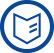Mission

The project-oriented research at the Weierstrass Institute is characterized by combining the mathematical disciplines of analysis, stochastics and numerics. This combination has great potential for solving complex applied problems. The institute aids in solving current societal challenges, such as the reliable extraction of information from large datasets or the suitable consideration of uncertainties in describing processes.

more

Research

The institute dedicates itself to fundamental mathematical research as well as the development of algorithms and scientific software. During the problem-solving process, mathematical models of physical and technological systems are designed that properly capture observed phenomena, thereby providing access to highly developed mathematical analysis. At WIAS the phases of the solving process are repeated and coordinated until an optimal solution is found.

more

Mathematical Core Areas

The WIAS is organised in eight research groups specializing in different mathematical techniques and methods. Additional temporary teams are created as the need arises. The core areas of mathematical research are the analysis and numerics of partial differential equations, and stochastics.

more

Main Application Areas

Research at WIAS focuses on the following application areas

more

Cooperation and Consulting

Modern applied mathematical methods are a fundamental resource and driving force for technological and economic development around the world. The rapid development of computer technology and mathematically based numerical methods are making it possible to numerically simulate ever more complex engineering, medical, economic and environmental problems.

At the WIAS excellent fundamental research is combined with years of experience in cooperating successfully with partners in the widest possible mix of application fields. The institute is therefore a recognized expert in the solution of complex economic, scientific, and technical problems by means of mathematical modeling and numerical simulation.

moreNews

“SeMA Journal best paper award” to Volker John and coauthors
Scientific paper “A unified analysis of algebraic flux correction schemes for convection–diffusion equations” awarded by the Spanish Society of Applied Mathematics (SEMA).

more

Upcoming Event
Monday, 24.06.2019 (WIAS-ESH)
Workshop on Phase Transitions and Particle Systems (Workshop/Conference)

further eventsJobs

PhD Student Position (f/m/d) (19/11)
Development, implementation, and numerical analysis of advanced discretizations of partial differential equations

PhD Student Position (f/m/d) (19/12)
Interacting random systems; statistical physics; percolation; stochastic geometry

Studentische Hilfskraft (m/w/d) (19/13)
Unterstützung der Gruppe Rechentechnik bei der täglichen Arbeit